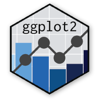Show Sidebar Hide Sidebar# geom_text in ggplot2

How to make a text graph using ggplotly.

### New to Plotly?

Plotly's R library is free and open source!
You can set up Plotly to work in online or offline mode.
We also have a quick-reference cheatsheet (new!) to help you get started!

### Version Check

Version 4 of Plotly's R package is now available!
Check out this post for more information on breaking changes and new features available in this version.

library(plotly)
packageVersion('plotly')

##  '4.9.1'


### Basic Text Graph

Sources: International IDEA for national turnout and European Parliament for European turnout, while regional classifications are based on EuroVoc.

recent_turnout <- read.csv("https://raw.githubusercontent.com/plotly/datasets/master/european_turnout.csv",stringsAsFactors = FALSE)
recent_turnout$region <- factor(recent_turnout$region, levels=c("British","Northern","Western","Mediterranean","Central/Eastern"))

library(plotly)
p <- recent_turnout %>%
ggplot(aes(x=nat_turnout,y=euro_turnout)) +
geom_text(aes(size=population/3.5, label=abbreviation, colour=region), alpha=1) +
labs(title = "Recent turnout in European Union countries",
x = "Latest legislative or presidential election (whichever had higher turnout)",
y = "May 2019 European Parliament election")
p <- ggplotly(p)

p


### Overlaid Points

Colour-coding the text itself might present readability issues. Another possible use of geom_text is to keep the text grey, but overlay it on a coloured point graph.

Adding the text option within aes() allows us to control the text that appears when hovering over a point.

recent_turnout <- read.csv("https://raw.githubusercontent.com/plotly/datasets/master/european_turnout.csv",stringsAsFactors = FALSE)
recent_turnout$region <- factor(recent_turnout$region, levels=c("British","Northern","Western","Mediterranean","Central/Eastern"))

library(plotly)
p <- recent_turnout %>%
ggplot(aes(x=nat_turnout,y=euro_turnout)) +
geom_point(aes(size=population, colour=region, text=paste("country:", country)), alpha=0.4) +
geom_text(aes(size=population/3.5, label=abbreviation), colour="gray20", alpha=1) +
labs(title = "Recent turnout in European Union countries",
x = "Latest legislative or presidential election (whichever had higher turnout)",
y = "May 2019 European Parliament election")
p <- ggplotly(p)

p


### Customed Colour and Size Scale

Let's use the LaCroixColoR package to spruce up the colour scheme. In addition, by using scale_size_continuous, we can make sure that none of the text is too small.

recent_turnout <- read.csv("https://raw.githubusercontent.com/plotly/datasets/master/european_turnout.csv",stringsAsFactors = FALSE)
recent_turnout$region <- factor(recent_turnout$region, levels=c("British","Northern","Western","Mediterranean","Central/Eastern"))

library(plotly)
library(LaCroixColoR)
p <- recent_turnout %>%
ggplot(aes(x=nat_turnout,y=euro_turnout)) +
geom_point(aes(size=population, colour=region, text=paste("country:", country)), alpha=0.4) +
geom_text(aes(size=population/3.5, label=abbreviation), colour="gray20", alpha=1) +
scale_colour_manual(values=lacroix_palette(n=6, name="PeachPear")) +
scale_size_continuous(range = c(3, 8)) +
labs(title = "Recent turnout in European Union countries",
x = "Latest legislative or presidential election (whichever had higher turnout)",
y = "May 2019 European Parliament election")
p <- ggplotly(p)

p


Adding a regression line as well as a label. geom_smooth does not allow for adjusting the transparency of the line (using alpha), which is why stat_smooth is used here. annotate is used to include a single text label (geom_text would create one label for every data point, all overlapped with each other).

recent_turnout <- read.csv("https://raw.githubusercontent.com/plotly/datasets/master/european_turnout.csv",stringsAsFactors = FALSE)
recent_turnout$region <- factor(recent_turnout$region, levels=c("British","Northern","Western","Mediterranean","Central/Eastern"))
m <- lm(euro_turnout ~ nat_turnout, data = recent_turnout)

library(plotly)
library(LaCroixColoR)
p <- recent_turnout %>%
ggplot(aes(x=nat_turnout,y=euro_turnout)) +
stat_smooth(geom="line", method="lm", alpha=0.3, se=FALSE) +
geom_point(aes(size=population, colour=region, text=paste("country:", country)), alpha=0.4) +
geom_text(aes(size=population/3.5, label=abbreviation), colour="gray20", alpha=1) +
scale_colour_manual(values=lacroix_palette(n=6, name="PeachPear")) +
scale_size_continuous(range = c(3, 8)) +
labs(title = "Recent turnout in European Union countries",
x = "Latest legislative or presidential election (whichever had higher turnout)",
y = "May 2019 European Parliament election") +
annotate(geom="text", x=60, y=80, label = paste("European turnout = \n",
round(unname(coef(m)),2),
"x national turnout",
round(unname(coef(m)),1)))
p <- ggplotly(p)

p


### Customized Formatting

Changed the font of the geom_text and of the graph (these must be done separately!), corrected the size label, centre-aligned the title.

recent_turnout <- read.csv("https://raw.githubusercontent.com/plotly/datasets/master/european_turnout.csv",stringsAsFactors = FALSE)
recent_turnout$region <- factor(recent_turnout$region, levels=c("British","Northern","Western","Mediterranean","Central/Eastern"))
m <- lm(euro_turnout ~ nat_turnout, data = recent_turnout)

library(plotly)
library(LaCroixColoR)
p <- recent_turnout %>%
ggplot(aes(x=nat_turnout,y=euro_turnout)) +
stat_smooth(geom="line", method="lm", alpha=0.3, se=FALSE) +
geom_point(aes(size=population, colour=region, text=paste("country:", country)), alpha=0.4) +
geom_text(aes(size=population/3.5, label=abbreviation), colour="gray20", alpha=1, family="Fira Sans") +
scale_colour_manual(values=lacroix_palette(n=6, name="PeachPear")) +
scale_size_continuous(range = c(3, 8)) +
labs(title = "Recent turnout in European Union countries",
x = "Latest legislative or presidential election (whichever had higher turnout)",
y = "May 2019 European Parliament election",
size = "") +
annotate(geom="text", x=60, y=80, label = paste("European turnout = \n",
round(unname(coef(m)),2),
"x national turnout",
round(unname(coef(m)),1))) +
theme(plot.title = element_text(hjust = 0.5)) +
guides(size=guide_legend(""), fill = FALSE) +
theme(text = element_text(family = 'Fira Sans'))
p <- ggplotly(p)

p